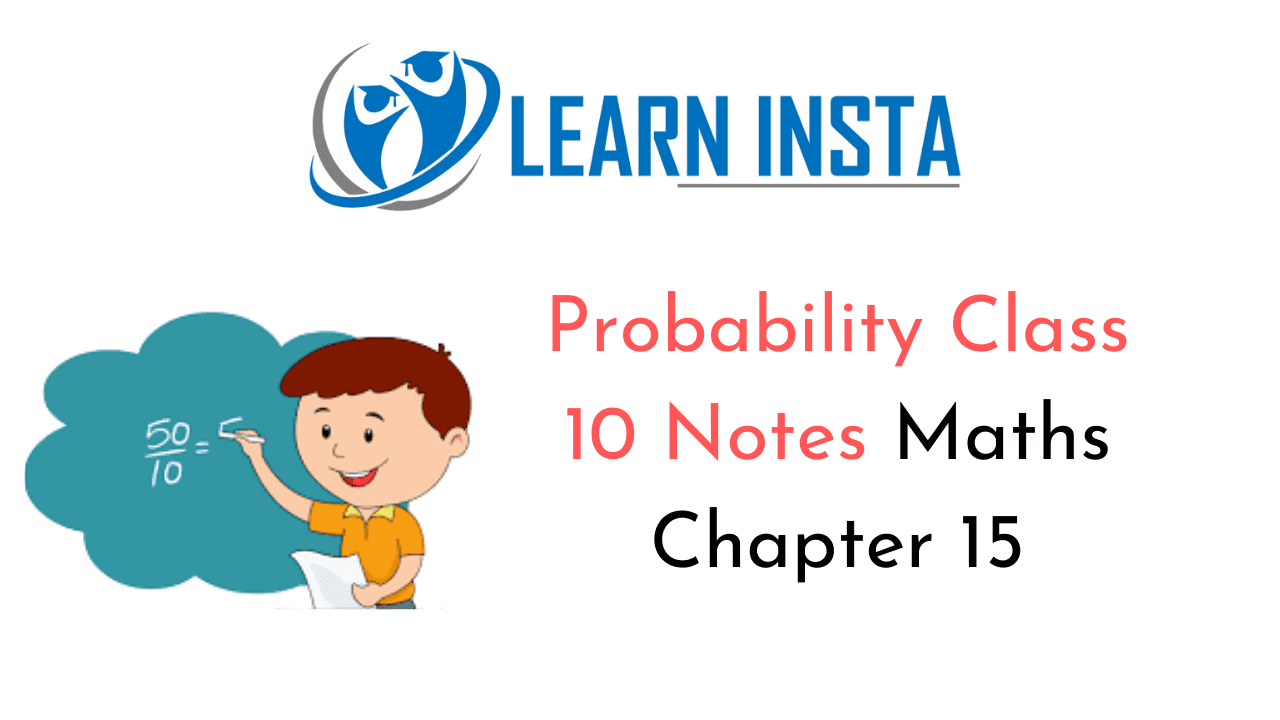On this page, you will find Probability Class 10 Notes Maths Chapter 15 Pdf free download. CBSE NCERT Class 10 Maths Notes Chapter 15 Probability will seemingly help them to revise the important concepts in less time.

## CBSE Class 10 Maths Chapter 15 Notes Probability

### Probability Class 10 Notes Understanding the Lesson

Theoretical probability: The theoretical (or classical) probability of an event E [denoted by P(E)] is given by
$$\mathrm{P}(\mathrm{E})=\frac{\text { Number of outcomes favourable to } \mathrm{E}}{\text { Number of all possible outcomes of the experiment }} \text { i.e., } \frac{n(\mathrm{A})}{n(\mathrm{S})}$$

Number of all possible outcomes of the experiment when the outcomes of the experiment are equally likely.

Equally likely outcomes: All the outcomes of an experiment are said to be equally likely when the chances of there occurrence are equal.
e.g. When a coin is tossed, the two possible outcomes are head and tail, which are equally likely.

Elementary event: An outcome of a random experiment is called an elementary event. e.g. In tossing a coin, possible outcomes are head and tail.
⇒ H and T are elementary events.

• The sum of the probabilities of all the elementary events of an experiment is 1.
• For an events E, P(E) + P($$\overrightarrow{\mathrm{E}}$$) = 1, where $$\overrightarrow{\mathrm{E}}$$ is the event ‘Not E’. E and $$\overrightarrow{\mathrm{E}}$$ are called complementary events.
• If P(E) = 1, then E is called ‘sure or certain event’.
• If P(E) = 0, then E is impossible event.
• For any event E,
0 < P(E) <1